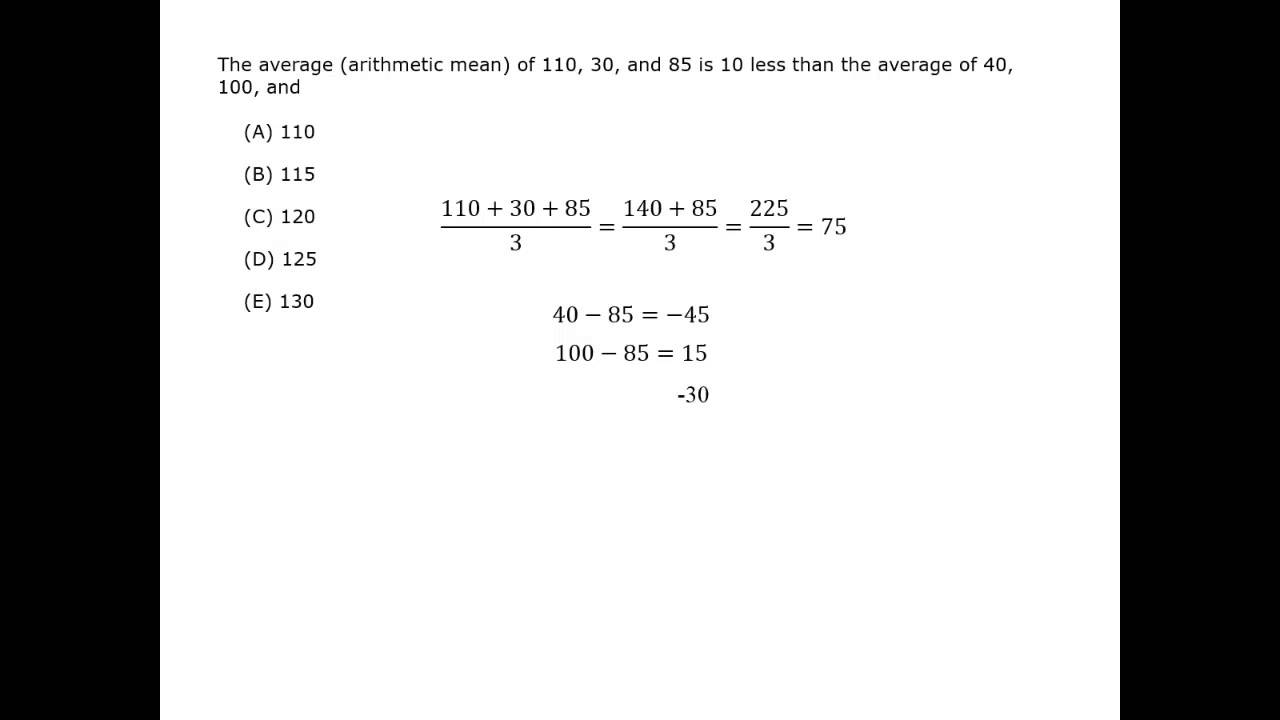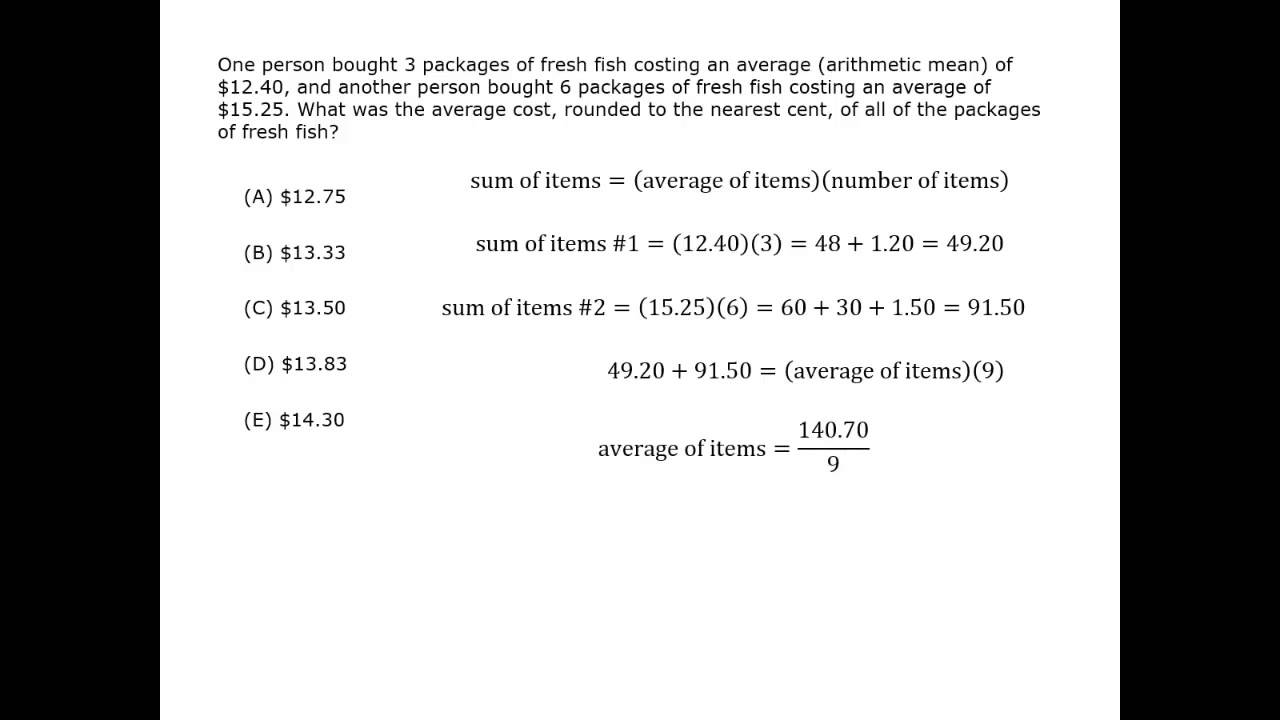1. Averages

An average is a number used to describe a set of data. Averages give a measure of the “middle” of a set.

The most common averages are the arithmetic mean, the median and the mode. The mean is often thought of as the average, but the terms are not interchangeable. The GMAT uses the term arithmetic mean instead of average to be more precise.

### 2. Arithmetic Mean and Median

The arithmetic mean is the sum of a set of numbers divided by the number of elements in the set.

mean = \dfrac{sum \,of \,the \,values}{number \,of \,values}

The median is the “middle” number of a list of data. To find the median, arrange the numbers in numerical order. If the number of values is odd, the median is the middle number. If the number of values is even, the median is the mean of the two middle numbers.

The median can differ greatly from the arithmetic mean.

Using the median, half of the people in a neighborhood pay more than the median rent, and half pay less. For example, if 5 people have monthly rent of $1100,$900, $1000,$10,000 and $3000, the median is$1100.

The mean does not split the people into a top half and bottom half. For example, for the monthly rents given above, the mean rent is $3200. Compare this median of$1100 and the mean of $3200. If a large number of people pay very little rent and a few pay a huge amount, the mean would be quite high, but the median would be surprisingly low. #### Video Quiz #### Mean, Median and Mode Best viewed in landscape mode 5 questions with video explanations 100 seconds per question### 3. Mode and Range Two other values can be used to describe a set. The mode is the most frequently occurring number in a set of data. There can be more than one mode. {1, 2, 3, 3, 4, 5} mode = 3 {1, 2, 3, 3, 4, 5, 5} modes = 3 and 5 The mode has the feature that it can be used to describe things other than numbers that are the most frequently occurring (such as white trucks). The range isn’t an average. It describes the spread or dispersion of a data set. The range is the difference between the largest and smallest values. range = (greatest value) – (least value) Find the arithmetic mean, median and range.(a) 2, 6, 10, 20, 20, 26 (b) 5, 45, 15, 10, 5 ### Solution (a) mean = 2 + 6 + 10 + 20 + 20 + 26/6 = 14 The number of values is even, so the median is the mean of the two middle numbers. median = (10 + 20)/2 = 15 range = 26 – 2 = 24 (b) mean = 5 + 45 + 15 + 10 + 5/5 = 16 Putting the values in numerical order makes it easier to see the median and range. 5, 5, 10, 15, 45 The number of values is odd, so the median is the middle number. median = 10 range = 45 − 5 = 40 Victor earned scores of 70, 75, 80, 85, 85 and 90 on his first six algebra tests. What is the relationship between the averages of these scores?(A) median < mode < mean (B) median < mean < mode (C) mode < median < mean (D) mean < mode < median (E) mean < median < mode ### Solution mean = (70 + 75 + 80 + 85 + 85 + 90)/6 = 485/6 ≈ 80.8 median = (80 + 85)/2 = 82.5 mode = 85 80.8 < 82.5 < 85, so mean < median < mode. The correct answer is choice (E). Set P = {a, a, a, a, b, b, b}. If a < b, is the mean greater than the median? ### Solution The median is the middle value, so the median is a. mean = (4a + 3b)/7 The question is about the relationship between the expressions. median ? mean 7a ? 4a + 3b 3a ? 3b a ? b Since a < b was given and the median is a, the mean is greater than the median. ### 4. Comparing Data Every set of numbers has a mean, median and range. These three values can be used to compare data by seeing how much the numbers differ. A politician announces at a press conference that the average yearly salary per person in the state is now$80,000.After her announcement, three groups of 5 people were surveyed about their salaries.

(I) $80,000,$80,000, $80,000,$80,000, $80,000 (II)$71,000, $74,000,$82,500, $82,500,$90,000
(III) $15,000,$27,000, $33,000,$100,000, $225,000 Is the claim about average salaries reasonable for each group? ### Solution To solve this problem, compare the mean, median and range of each data set. (I) The mean and median are$80,000. The range is 0. The claim is reasonable for this group.

(II) The mean is $80,000. The median is$82,500. The range is $19,000. So the mean and median support the claim. The range is somewhat high, showing the data is spread out. The claim is still somewhat reasonable for this group. (III) The mean is$80,000. The median is $30,000. The range is$210,000. The claim is not reasonable for this group.

The mean age of a group of 10 firefighters is 18 years. If 2 firefighters join the group, the mean age increases by 2 years. What is the mean age of the new firefighters?(A) 18
(B) 20
(C) 24
(D) 30
(E) 60

### Solution

The mean age of the first 10 firefighters is 18, so the total of their ages is 10 × 18 = 180.

The mean age of the 12 firefighters is 18 + 2 = 20, so the total of their ages is 12 × 20 = 240.

The difference between the 2 totals is the total for the ages of the 2 new firefighters.
240 – 180 = 60

So the mean of the ages of the 2 added firefighters is 60 / 2 = 30.

The correct answer is choice (C).

Monica drove for 2 hours at 45 miles per hour and 3 hours at 60 miles per hour. What was her average (arithmetic mean) speed for the whole trip?

### Solution

The formula is distance = rate × time, or d = rt. You want to find the rate.

distance = (2 × 45) + (3 × 60) = 270 miles
time = 2 + 3 = 5 hours

So 270 = 5r, and r = 54. Her average speed was 54 miles per hour.

Notice that the average speed is not (45 + 60)/2 = 52.5

The average rate = (total distance) / (total time)

Set S consists of 5 consecutive integers. Set P is created by increasing the value of each element in set S by 2 times its place in S. (The value of the first element in S is increased by 2, the value of the second element by 4, and so on.) How much greater is the mean of set P than the mean of set S?(A) 3
(B) 5
(C) 6
(D) 10
(E) 30

### Solution

The question here is the change in the mean. The change in the mean will be the sum of each of the changes divided by the number of changes.

mean of changes = (2 + 4 + 6 + 8 + 10) / 5 = 6

So the mean of set P is 6 greater than the mean of set S.

The correct answer is choice (C).

Set S = { 1/2 , –x , x , x2 , -2x , 2x }. If – 1/2 < x < 0, what is the median of set S?(A) 1/4 – x/2

(B) x2/2 – 1/4

(C) x(x – 1)/2

(D) 1/2x

(E) x(x + 1)/2

### Solution

Method 1

Since -1/2 < x < 0, the simplest technique is to Plug In: substitute a number to find a reasonable value. Use x = -1/4. Then the values are:

1/2

x = -(-1/4) = 1/4

x = -1/4

x2 = (-1/4)2 = 1/16

-2x = -2(-1/4) = 1/2

2x = -1/2

Now arrange the data in numerical order. Since there are 6 elements in the set, you are looking for the 2 middle values.

-1/2, -1/4, 1/16, 1/4, 1/2, 1/2

Using the values, the median is (1/16(1/16) + (1/4)/2 .

So using the variables, the median is x2 + (-x)/2 = x2x/2 = x(x – 1)/2 .

The correct answer choice is (C).

Method 2

First arrange the elements in numerical order.

Because -1/2 < x < 0:

2x and x are negative and 2x < x.
x2 and –x are positive and x2 < –x < 1/2.

It is not clear whether 1/2 < -2x. But it is not important as long as we know that –x and x2 are the third and fourth largest elements in the set (because there are six total elements in the set).

So, the elements in numerical order will either be {2x, x, x2, –x, 1/2, 2x} or {2x, x, x2, –x, 2x, 1/2} With six elements in the set, the median will be the mean of the two middle values of the set.

median = x2x/2 = x(x – 1)/2

The correct answer choice is (C).

#### Mean, Median and Mode

Best viewed in landscape mode

5 questions with video explanations

100 seconds per question